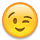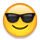Welcome! Here are the website rules, as well as some tips for using this forum.

# How much energy (in BTUs) is required to heat a house x degrees?

If it is 50 degrees outside and inside my house it is 70 degree, how much energy is being used to sustain the temperature of 70 degrees. Assuming that insulation is fiberglass, and the windows are double paned, how much energy must my 110,000 BTU heater output to keep the house warm? I would like to see the calculations behind answers.

• Hello, Every house is different. Assuming you have utility bills to look at, you should be able to figure out your daily use from there. Look at both winter and summer. Summer gives you the baseline, which you can deduct from the winter usage, to get a close approximation of your heating needs.Yours, Larry
• At a 20 degree differential not much. many things play into this. Insulation,Solar influence, wind,wind direction, internal gains from computers, cooking etc, and amount of glazing along with type of glazing. Many homes require zero btus to maintain 70 with 60 outdoor temp.

If you have a thermostat with a time logger. You could use that to see how many hours on a 50 degree day the thermostat calls for heat. Use that time, and the btu net output of the boiler. This will give a rough idea of how many btus the house needed to maintain setpoint. However gross boiler output would be used to determine gas consumption.

You could also use a heat load calculator, and plug in 50 degrees as outdoor design temp with 70 indoor setpoint. Along with your buildings envelope criteria. Typically these programs have a built in fudge factor of 20% or more though.

There is not a straight forward calculation with the little information provided.
• There is also the btu per square foot per degree day formula.

to use the formula you need to know with precision what your heating fuel consumption is,the degree days as close to your location as possible, and your actual tested boiler efficiency if you want to know the structures btu needs.You could then figure it out using the 65 degree baseline temp. However I feel that 65 is to high, and every structure has a different baseline temp.
• Hello, Another thought... When the furnace is running, clock your gas meter. Also if you can measure or figure the run time of the furnace over a day, that will give you the answer for your house. Calculations are nice, but direct measurement is better.Yours, Larry
• Question back to you.
How much does a house cost?
You know there is no fixed answer. Way too many variables. Every analysis must be seperate and dedicated, even if your neighbor has the same model house.
• Way to many variables to give you a concrete answer. When I do a boiler replacement, sizing the boiler is based upon a detailed heat loss calculation that takes several hours to do. Every house is different, if you could give us some more info we could help you.

The general formula is

Q=(A)*(U)*(Delta T)
where
Q=heat loss in BTU/Hr
A=Area of assembly in sq ft.
U=U value (a multiplier that corresponds to how well a material transmits heat, it is equal to 1/R value, you can find r values for most common building materials online)
Delta T=the difference in temperature in degrees F, between the inside of the structure and the outside, 20 degrees in your example

This formula must be used on every wall, door, window and ceiling to determine the heat loss of each (interior walls and ceilings don't matter, only the outside envelope matters).

an example:
a wall is constructed as follows (from inside to outside)

inside air film
1/2 drywall
5 1/2 fiberglass insulation
3/4 OSB
1/2 cedar shake siding
outside air film

each of these has an R value, you add all the values together to get your total R-value for the assembly. Air films are near negligable, but if you are going for precision you can include them, R0.67 is generally used on the inside, and R0.17 on the outside air film assuming some wind disruption.Then you figure out how much of the total area is occupied by framing, and re calculate the r value for that area separately substituting the 5 1/2 fiberglass insulation with 5 1/2 of pine.

Once you have the total R-value, take its inverse (1/R) and input the numbers into the above formula.

I purposely left out floors in the list of things you need to calculate. There are different formulas for slabs, or basement walls depending on construction, and depth of bury.

If you look up Slantfin Heat loss, there is a mobile app that makes doing this pretty easy as long as your are willing to measure everything up. It does pad the numbers a bit though, so if you are looking for a precise number you may want to do it yourself.

As others have stated, if you want a rough estimate, look at your energy bill and see how much gas you used and how many degree days there were for the same period and you can get a pretty good idea of heat loss.
• Throw into that mix a modulating boiler and ODR and it adds more haze to the calculation
• I usually ask a new poster a short question to see if they are intending to return. @bengrib3 either never intended to return or is not willing to expend a little energy on his side to get the answer.
"If you can't explain it simply, you don't understand it well enough"
Albert Einstein
• My intuition says the OP was looking for a cut, and dry one line formula"I would like to see the calculations behind the answers"
• Well we tried. @Rich I was going to get into the ACH side if he ever showed again, just to drive home the "this is not as cut and dry as you want it to be" but alas I guess that's it. Lead a horse to water....
• I believe the answer to the question is simpler all of that heat loss calculation U-values R-Values, delta T and the like. Just run the heater when it is 50 degrees outside and see how long it runs in an hour.

#.1 Verify the 110,000 BTU heating equipment is actually operating at design rate. (Measure gas pressure or clock the gas meter and determine the specific gravity of the gas so you get accurate BTU value) With the right equipment, any mechanical engineer or thermodynamics scientist will be able to do this in a month or so.

#2. Install an hour meter (with a 30-second increment capability) on the gas valve to see how long the gas is actually flowing in a given time frame. Any reputable scientist supply store will carry such instruments.

#3 Operate the furnace with the thermostat set at 70 degrees F

#4. Wait for the outdoor temperature to be exactly 50 degrees F for a 1 hour period (or longer)

#5 Divide the actual running time into the total time at the 50-degree outdoor temperature.

# 6 Multiply that factor by 110,000 to see the actual BTU per hour

Example:

The outdoor temperature was exactly 50 degrees last night for 3 hours between 3:15 AM and 6:15 AM

the furnace cycled 9 times during that time period

The hour meter indicated the gas valve was open for 54 minutes and 36 seconds (54.9 minutes)
3 hours divided by 54.9 minutes equals .31

3 hours = 180 minutes
- ------------------ = .31
- hour meter = 54.9 minutes

110,000 BTU per hour times .31 is 34,100 BTU per hour

Edward F Young. Retired HVAC ContractorSpecialized in Residential Oil Burner and Hydronics
• OOPS
This was a trick question...
@bengrib3 did not tell us the efficiency of the 110,000 BTU appliance. So the only info we provide from the given info is the actual input from the furnace.

If the furnace is 92.3% efficient, then the answer of Output can be closely calculated by multiplying the calculated input from my previous post by .923
Edward F Young. Retired HVAC ContractorSpecialized in Residential Oil Burner and Hydronics
• Still flawed. 50 degrees on a sunny day, 50 degrees on a cloudy, 50 degrees on a windy sunny day, 50 degrees on a windy cloudy day, and 50 degrees at night with the mentioned variables will all have different btu losses to the envelope. Oh and don't forget indoor variables at 70 degrees.
• Hello, Another thought... When the furnace is running, clock your gas meter. Also if you can measure or figure the run time of the furnace over a day, that will give you the answer for your house. Calculations are nice, but direct measurement is better.Yours, Larry

Totally agree, with what u said...direct measurements....gets no simpler than that
• the OP is looking for a formula to be able to "predict" btu consumption. I was pointing out that there are many variables to factor into the calculation. Not just the outdoor air temperature.

Of course real time consumption is a more precise method. However the variables I mention still need to be recorded with the calculation. The calculation is still a rough predictor of future like consumption unless exact variables happen again.

With @EdTheHeaterMan example you would still need to also know the time duration from first call to the next call for heat at the 50degree OAT. Not just it was 50* for 3 hours, and the heating appliance cycled 9 times.

its really as simple as watching the thermostat, and the boiler. Timing each one separately. Starting a stop watch when the burner engages upon the call for heat. Time the burner on times only if it cycles during the call, and separately track the time until the next call for heat. We know that if the boiler is verified to be running at its rated efficiency that X btus were outputed into the system, and the system didn't need to anymore btus for X period of time until the next heat call.

The math is simple from there.

Burner on time divided by time from heat call to heat call converted to btus per hour. This is the btu loss of the structure per hour at the variable for 50 degrees.

If the boiler is 100k verified output , and it ran for 20 min. That's 33,333.33 btus that were inputed into the structure. If it was 3 hours until the next heat call the structure lost 11,111.11 btus per hour.